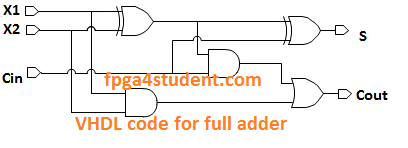# 完整加法器的VHDL代码

## 在这方面 VHDL项目 ，VHDL代码 完整的加法器 被表达。通过使用行为和结构模型实现加法器的VHDL代码。

#### 完整的加法器有三个输入X1，X2，随身携带的CIN和两个输出S，执行型COUT，如下图所示：### 使用结构模型的完整加法器的VHDL代码：

```-- fpga4student.com
-- FPGA projects,  VHDL项目 , Verilog projects
-- 完整加法器的VHDL代码
-- Structural code for full adder
library ieee;
use ieee.std_logic_1164.all;
entity Full_Adder_Structural_VHDL is
port(
X1, X2, Cin : in std_logic;
S, Cout : out std_logic
);
end Full_Adder_Structural_VHDL;
architecture structural of Full_Adder_Structural_VHDL is
signal a1, a2, a3: std_logic;
begin
a1 <= X1 xor X2;
a2 <= X1 and X2;
a3 <= a1 and Cin;
Cout <= a2 or a3;
S <= a1 xor Cin;
end structural;
Library IEEE;
USE IEEE.Std_logic_1164.all;
-- fpga4student.com
-- FPGA projects, VHDL projects, Verilog projects
-- 完整加法器的VHDL代码
-- Testbench code of the structural code for full adder
entity Testbench_structural_adder is
end Testbench_structural_adder;

architecture  behavioral of Testbench_structural_adder is
component Full_Adder_Structural_VHDL
port(
X1, X2, Cin : in std_logic;
S, Cout : out std_logic
);
end component;
signal A,B,Cin: std_logic:='0';
signal S,Cout: std_logic;
begin
structural_adder: Full_Adder_Structural_VHDL port map
(
X1 => A,
X2 => B,
Cin => Cin,
S => S,
Cout => Cout
);
process
begin
A <= '0';
B <= '0';
Cin <= '0';
wait for 100 ns;
A <= '0';
B <= '0';
Cin <= '1';
wait for 100 ns;
A <= '0';
B <= '1';
Cin <= '0';
wait for 100 ns;
A <= '0';
B <= '1';
Cin <= '1';
wait for 100 ns;
A <= '1';
B <= '0';
Cin <= '0';
wait for 100 ns;
A <= '1';
B <= '0';
Cin <= '1';
wait for 100 ns;
A <= '1';
B <= '1';
Cin <= '0';
wait for 100 ns;
A <= '1';
B <= '1';
Cin <= '1';
wait for 100 ns;
end process;

end behavioral; ```

#### 完整加法器结构VHDL代码的模拟波形：### 使用行为模型的完整加法器的VHDL代码：

``` -- fpga4student.com
-- FPGA projects, VHDL projects, Verilog projects
-- 完整加法器的VHDL代码
-- Behavioral code for full adder
library ieee;
use ieee.std_logic_1164.all;
use IEEE.STD_LOGIC_unsigned.ALL;
use IEEE.NUMERIC_STD.ALL;
entity Full_Adder_Behavioral_VHDL is
port(
X1, X2, Cin : in std_logic;
S, Cout : out std_logic
);
end Full_Adder_Behavioral_VHDL;
architecture Behavioral of Full_Adder_Behavioral_VHDL is
signal tmp: std_logic_vector(1 downto 0);
begin
process(X1,X2,Cin)
begin
tmp <= ('0'& X1) + ('0'& X2) +('0'& Cin) ;
end process;
S <= tmp(0);
Cout <= tmp(1);
end Behavioral;
Library IEEE;
USE IEEE.Std_logic_1164.all;
-- fpga4student.com
-- FPGA projects, VHDL projects, Verilog projects
-- 完整加法器的VHDL代码
-- Testbench code of the behavioral code for full adder
entity Testbench_behavioral_adder is
end Testbench_behavioral_adder;

architecture  behavioral of Testbench_behavioral_adder is
component Full_Adder_Behavioral_VHDL
port(
X1, X2, Cin : in std_logic;
S, Cout : out std_logic
);
end component;
signal A,B,Cin: std_logic:='0';
signal S,Cout: std_logic;
begin
behavior_adder: Full_Adder_Behavioral_VHDL port map
(
X1 => A,
X2 => B,
Cin => Cin,
S => S,
Cout => Cout
);
process
begin
A <= '1';
B <= '1';
Cin <= '1';
wait for 50 ns;
A <= '1';
B <= '1';
Cin <= '0';
wait for 50 ns;
A <= '1';
B <= '0';
Cin <= '1';
wait for 50 ns;
A <= '0';
B <= '0';
Cin <= '0';
wait for 50 ns;
A <= '0';
B <= '0';
Cin <= '1';
wait for 50 ns;
A <= '0';
B <= '1';
Cin <= '0';
wait for 50 ns;
A <= '0';
B <= '1';
Cin <= '1';
wait for 50 ns;
A <= '1';
B <= '0';
Cin <= '0';
wait for 50 ns;

end process;

end behavioral;
```

#### 完整加法器的行为VHDL代码模拟波形：